# PSEB 5th Class Maths Solutions Chapter 10 Data Handling Ex 10.1

Punjab State Board PSEB 5th Class Maths Book Solutions Chapter 10 Data Handling Ex 10.1 Textbook Exercise Questions and Answers.

## PSEB Solutions for Class 5 Maths Chapter 10 Data Handling Ex 10.1

Question 1.
The students of a school visited a zoo for picnic. Students collected the data of number of different animals. The number of animals are as follows : Monkey – 32, Lion – 10, Deer – 25, Rabbit – 27 and Fox – 39. Represent the data in tabular form.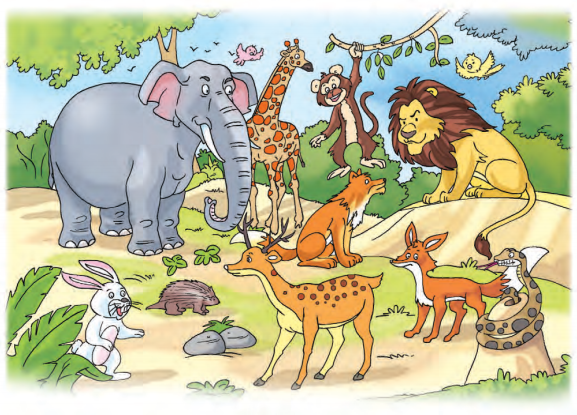Solution:
We can represent the above information in the tabular form as follows :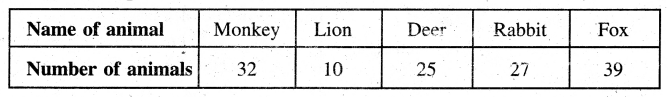Question 2.
There is a circus in a village. The following pictograph represents the number of children visitors from Monday to Friday. Read the following pictograph carefully and answer the questions.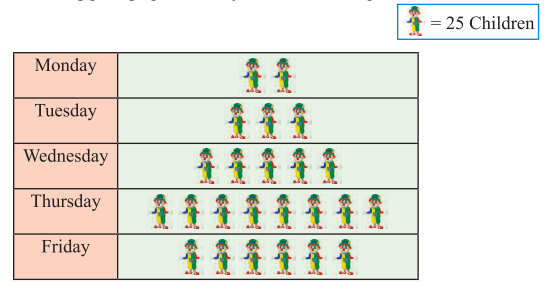1. How many children visited circus on Tuesday ?
2. Which day had most children visited and how many ?
3. Which day had least children visited and how many ?
4. How many total number of children visited the circus on Monday and Wednesday ?
5. What is the difference of number of children visited on Thursday and Friday ?

Solution:

1. Number of children who visited circus on Tuesday = 3 x 25 = 75.
2. The day on which the most number of the children had visited is Thursday and the number of children is 8 × 25 = 200.
3. The day on which least number of children had visited = Monday and the number of children = 2 × 25 = 50.
4. Total number of children who visited the circus on Monday and Wednesday = 50 + 125=175.
5. The difference of number of children who visited on Thursday and Friday = 200 – 150 = 50.Question 3.
The following table shows the plantation of trees on Diwali day in 5 different villages on the eve of celebration of Green Diwali :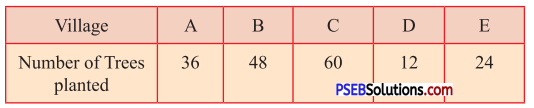(i) Draw pictograph of the above data.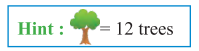Solution: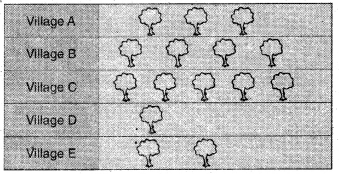(ii) Draw another pictograph of above information by any other scale.
Solution: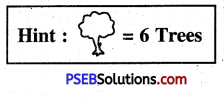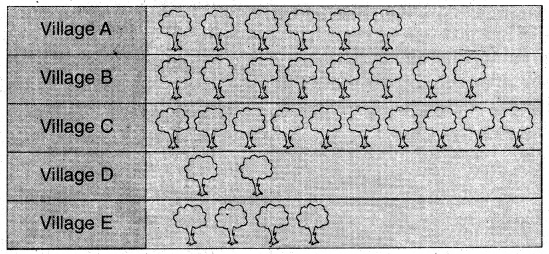Question 4.
The following Bar Graph represents the quantity of milk used in a week in a sweet shop during the festival season.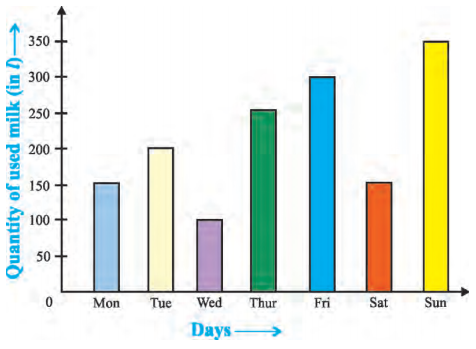1. On which day maximum milk is used ?
2. On which day minimum milk is used ?
3. On which two days, the quantity of milk used is the same and how much ?
4. How much total quantity of milk is used on Thursday and Friday ?
5. How much less milk is used on Tuesday than Sunday ?
6. What is the difference of maximum and minimum quantity of milk used ?

Solution:

1. Sunday
2. Wednesday
3. Monday and Saturday, 150 l
4. 550 l
5. 350 l – 200 l = 150 l
6. 350 l – 100 l = 250 l.Question 5.
The data of mobile phones sold of different companies of a shop in October is as follows :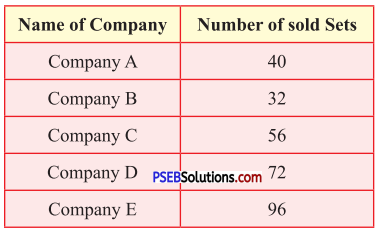Draw the bar graph for the above data.Scale : 8 sets = 1 unit
Solution: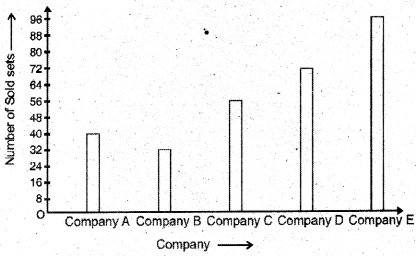Question 6.
The number of students from 1st to 5th class of a school is as follows: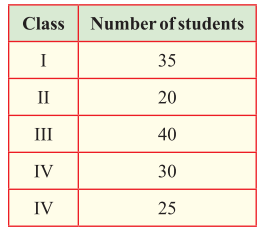Draw the bar graph for the above data.
Scale : 10 students = 1 unit
Solution: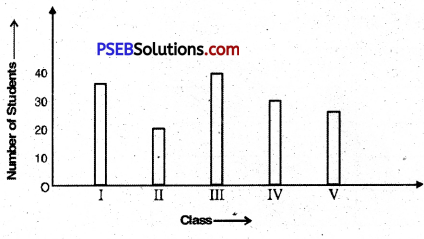Question 7.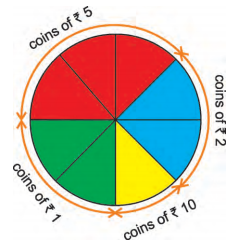The above Pie Chart is divided into 8 equal parts. It represents the different number of coins in Ajay’s Piggy Bank, then answer the following : If the number of total coins is 80.
(i) How many coins of ₹ 5 are there ? (In fraction)
(ii) How many coins of ₹ 2 are there ?
(iii) Tell the number of coins of ₹ 5 ?
(iv) What is the amount of coins of ₹ 10 ?
(v) How much total amount is there in Ajay’s Piggy bank ?
Solution:
(i) $$\frac{3}{8}$$
(ii) $$\frac{2}{8}$$ × 80 = 20
(iii) $$\frac{3}{8}$$ × 80 = 30

(iv) Number of ₹ 10 coins = $$\frac{1}{8}$$ × 80 = 10
Total value of ₹ 10 coins
= ₹ 10 × 10 = ₹ 100

(v) Number of ₹ 1 coins = $$\frac{2}{8}$$ × 80 = 20
Number of ₹ 2 coins = 20
Number of ₹ 5 coins = 30
Number of ₹ 10 coins = 10
Total value of ₹ 1 coins = ₹ 1 × 20 = ₹ 20
Total value of ₹ 2 coins = ₹ 2 × 20 = ₹ 40
Total value of ₹ 5 coins = ₹ 5 × 30 = ₹ 150
Total value of ₹ 10 coins = ₹ 10 × 10 = ₹ 100
The total amount of money in Ajay ’ s piggy bank
= ₹ 20 + ₹ 40 + ₹ 50 + ₹ 100
= ₹ 310.Question 8.
Given pie chart is divided into 12 equal parts. It represents the favourite festivals of 120 students of a school. Read it carefully and answer the following :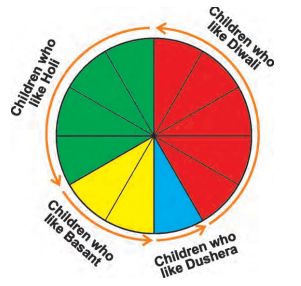1. How many students (in fraction) like Diwali festival ?
2. How many students like Holi festival out of 120 students ?
3. Which festival is liked by minimum number of students ?
4. What is the difference of number of students who like Diwali and Basant ?

Solution:

1. $$\frac{5}{12}$$
2. $$\frac{4}{12}$$ × 120 = 40
3. Dussehra
4. ($$\frac{6}{12}$$ × 120) – ($$\frac{2}{12}$$ × 120)
= 60 – 20 = 30